#### Study of Logic Gates

Introduction and Verification of Logic Gates:

To study two inputs basic gates such as NOR, NAND ,EX-OR, using TTL and IC and Verify the truth tables.

Components Required:

 S. no. Name of Components/Apparatus Quantity 1. 2 3. 4. 5. 6. DC regulated Power supply (0-5V) NOR Gate 7402 NAND Gate 7400 EXOR Gate 7486 LED Bread board 1 1 1 1 10 1

Procedure:

1. Fit the IC on the bread board

2. Switch on the power supply.

3. Inputs are given to the IC Input pins as per the schematic diagram and outputs are observed and tabulated for various logic gates.

4. 5V DC is set as 1 and 0 volt is 0.The inputs are mentioned as A and B.

5. Switch off the supply and remove the connections

1. NOR GATE:

It is a combination of NOT and OR gate and the operation of NOR gate is inverse of the OR gate.

1. Boolean equation for NOR gate is Y = A+B

2. If both the inputs of A or B = 0 then the O/P of Y = 1.

3. We can verify the operation by the truth tables and also by glowing of LED.

4. If the I/p is low then the o/p will be high.

5. Operation of the NOR gate is verified from the truth table.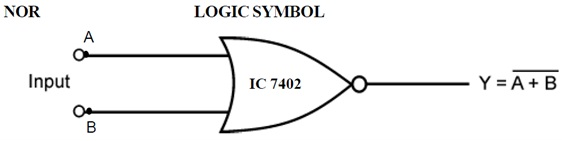NOR GATE CIRCUIT DIAGRAM: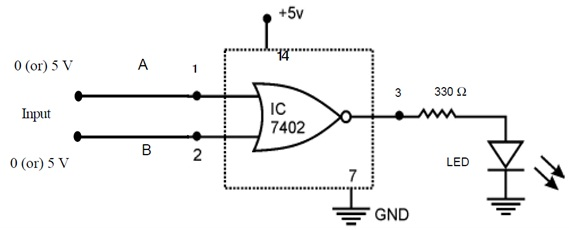NOR GATE PIN DIAGRAM OF IC - 7402: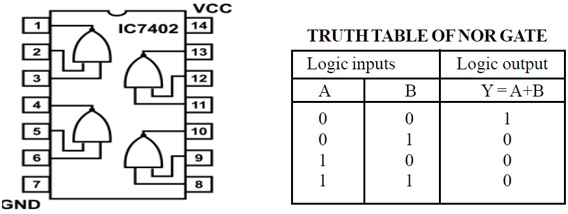NAND GATE                         LOGIC SYMBOL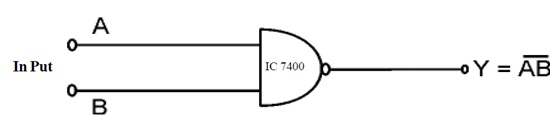NAND GATE CIRCUIT DIAGRAM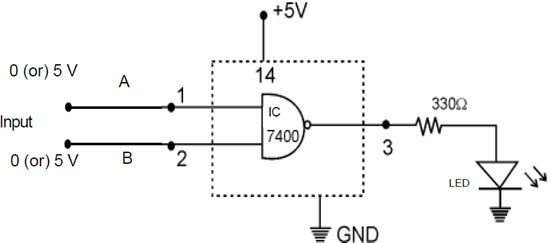IC 7400 PIN DIAGRAM: NAND GATE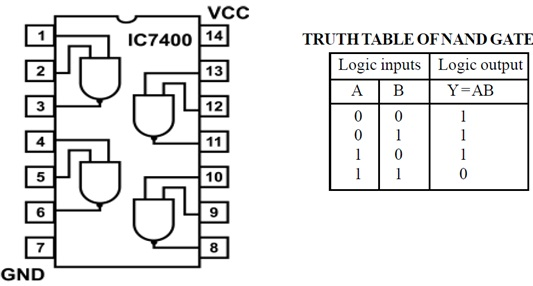EX - OR GATE                                            LOGIC SYMBOL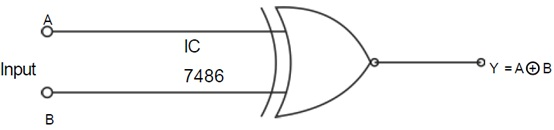CIRCUIT DIAGRAM EX - OR GATE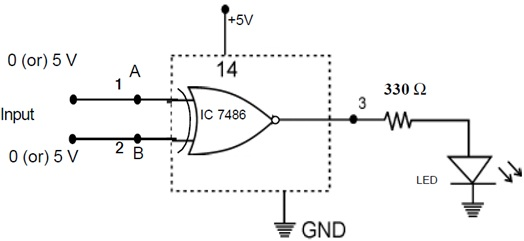IC 7486 PIN DIAGRAM OF EX - OR GATE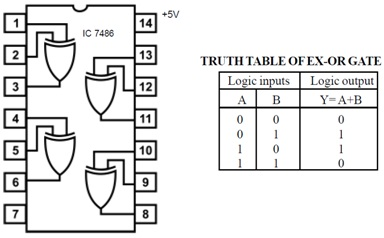NAND GATE:

Combination of nor and AND gate is called NAND gate It is a Reverse operation of AND gate.

1. Boolean equation of NAND gate is Y = AB

2. Inputs of A, B is O (or) any one of the input is one and another is 0 means the o/p will be 1.

3. Operation of the NAND gate is verified from the truth table

EX - OR GATE:

i) Diagram of Ex - OR gate shown using IC7486

ii) Boolean equation for Ex- or gate Y= A + B = AB + AB

iii) Any one of the I/P is high mean o/p will be high.

iv) It is verified from the truth table

Latest technology based Electrical Engineering Online Tutoring Assistance

Tutors, at the www.tutorsglobe.com, take pledge to provide full satisfaction and assurance in Study of Logic Gates homework help via online tutoring. Students are getting 100% satisfaction by online tutors across the globe. Here you can get homework help for Study of Logic Gates, project ideas and tutorials. We provide email based Study of Logic Gates homework help. You can join us to ask queries 24x7 with live, experienced and qualified online tutors specialized in Study of Logic Gates. Through Online Tutoring, you would be able to complete your homework or assignments at your home. Tutors at the TutorsGlobe are committed to provide the best quality online tutoring assistance for Electrical Engineering homework help and assignment help services. They use their experience, as they have solved thousands of the Electrical Engineering assignments, which may help you to solve your complex issues of Study of Logic Gates. TutorsGlobe assure for the best quality compliance to your homework. Compromise with quality is not in our dictionary. If we feel that we are not able to provide the homework help as per the deadline or given instruction by the student, we refund the money of the student without any delay.